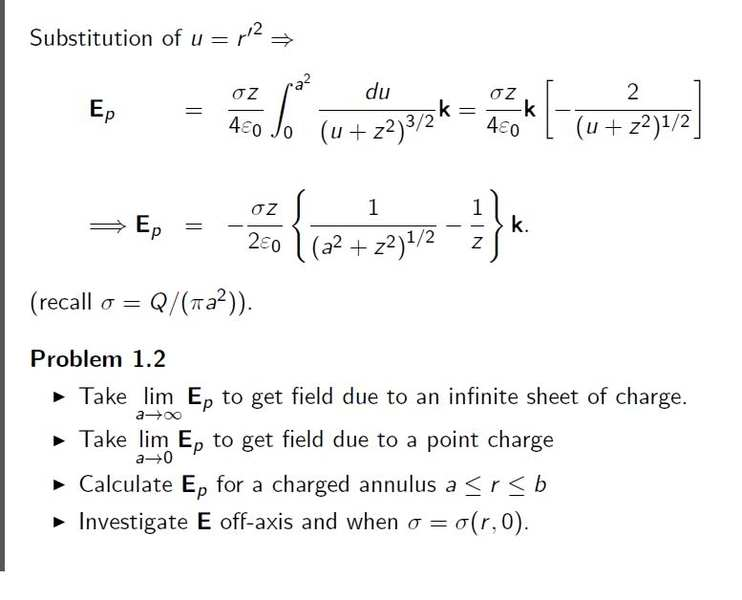# Electric field of a charged disc

... with constant charge density σ = Q/((pi)a^2)

The Electric field is, after some calculation, is given by E_p below:z is the z-axis, and a is the radius of the disc.

Now for the questions at the bottom of the page, here are my thoughts:

σ is independent of a because as a->2a, Q->4Q, and a^2 -> 4a^2. Same for all changes of scale.

As a -> infinity, E_p -> σ/(2ε_0)

But the second one I'm not sure about.

As a -> 0, surely the charge density stays constant i.e. at σ. Thus the field appears to be the 0 vector. However, we know that the field due to a point charge is proportional to r^/r^2, where r^ is the outward/inward unit vector and r is it's magnitude.

I know our area tends to 0 as a -> 0, so our charge Q must tend to 0 also. But this means our field from the "point charge" must be 0, disagreeing with the usual electric field for a point charge...

Philip Wood
Gold Member
Regarding the second question, you've thrown away the baby with the bath-water, when taking your limit. You need to work to first order in (a/z)2...

$$\frac{1}{\left(z^2+a^2\right)^\frac{1}{2}} = \frac{1}{z}\left(1+\frac {a^2}{z^2}\right)^{-\frac{1}{2}}\approx\frac{1}{z}\left(1-\frac {a^2}{2z^2}\right).$$

Make this substitution, and you'll finish up with the usual equation for the field due to a point source.

Last edited:
Right. At first I tried taylor series at x=0 but didn't try it at x = infinity. I guess I hadn't come across using expansion about infinity in physics yet... only complex analysis.

Maybe there is something simple I am missing but why do you automatically look for expansion about infinity?

Attempt to answer own question: because we are taking limit of a tending to 0. Expansion about z = 0 is a polynomial in z with a^i on the bottom, so the expansion will be something like infinity - infinity + infinity - ... which won't help us. Using expansion about infinity, we get a^i's on top and so the limit as a -> 0 is valid and useful. Amirite?

Also, your ways works because the 1/z's cancel, giving the simple answer, as opposed to a polynomial in z, where we can't make progress without taking steps backwards...

Last edited:
Philip Wood
Gold Member
I'm not regarding it as an expansion about infinity. I'm regarding (a/z), and therefore (a/z)2 as << 1, and expanding about (a/z) = 0. This amounts to using the binomial series (a special Taylor series), which is what I'm quoting, to first order in (a/z)2.

Last edited:
I'm not sure I get that at all. Are you sure you're not considering (a/z)^2 and therefore (a/z)^4 << 1 and similar for higher order terms? Because then the Binomial expansion formula would seem to work. I'm not used to using it with the exponent non-integer.

What I said in my last post is basically just writing out the Taylor series which is the same as the Binomial expansion because the Binomial expansion is the special case of Taylor we are dealing with...

Philip Wood
Gold Member
I am, as I said, taking the case of (a/z)<<1. This implies that (a/z)2<<<1 and (a/z)4<<<< 1, and so on. Therefore I'm neglecting all terms higher than the one in (a/z)2. It was one of Newton's discoveries that the binomial expansion also worked with non-integral indices, but went on for ever. It is extremely useful in Physics.
$$(1+x)^n = 1 + nx + \frac{n(n-1)}{2!}x^2 + \frac{n(n-1)(n-2)}{3!}x^3 ...$$

Last edited:
I see. Considering (a/z)<<1 or instead considering (a/z)^2<<1 leads to the same result (no information is lost w.r.t. the Binomial expansion approximation). But in both cases x = (a/z)^2